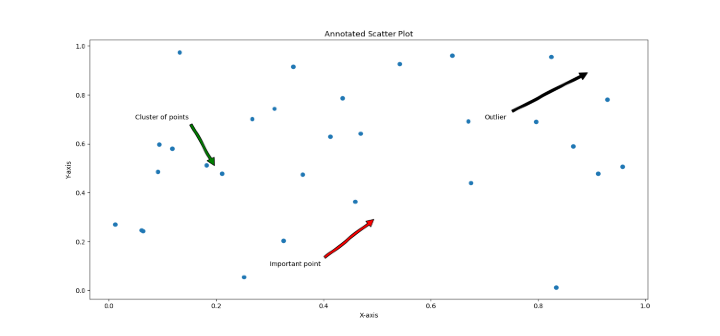# How to Annotate Matplotlib Scatter Plots?

## Introduction

Scatter plots are an essential tool for illustrating the connection between two continuous variables. They help us identify potential anomalies, patterns, and trends in the data. Yet, scatter charts can also be hard to interpret when there are numerous data points. If comments are made, some points of interest in a scatter plot could be easier to observe and understand. In order to make Matplotlib scatter plots more understandable, this article will examine how to annotate them.

## Syntax

ax.annotate(text, xy, xytext=None, arrowprops=None, **kwargs)

• text − Text to be displayed in the annotation.

• xy − (x,y) coordinates of the point to annotate.

• xytext − (x,y) coordinates of the text annotation. If None (default), xy is used as the text location.

• arrowprops − A dictionary of arrow properties. It specifies the style and color of the arrow connecting the text and the annotated point. Some commonly used properties include facecolor, edgecolor, arrowstyle, shrink, and width.

• **kwargs − Additional keyword arguments to be passed to the Text constructor.

Note − ax in the above syntax is the Axes object that is returned when creating a scatter plot in Matplotlib.

## Example

### Algorithm

• Import necessary libraries

• Create data points to be plotted

• Define the scatter plot using Matplotlib

• Add annotations to specific data points using text or arrow annotations

• Adjust the annotation formatting as needed

• Show the scatter plot with annotations

# Import necessary libraries
import matplotlib.pyplot as plt
import numpy as np

# Create data points to be plotted
x = np.random.rand(30)
y = np.random.rand(30)

# Define the scatter plot using Matplotlib
fig, ax = plt.subplots()
ax.scatter(x, y)

# Add annotations to specific data points using text or arrow annotations
ax.annotate('Outlier', xy=(0.9, 0.9), xytext=(0.7, 0.7),arrowprops=dict(facecolor='black', shrink=0.05))
ax.annotate('Important point', xy=(0.5, 0.3), xytext=(0.3, 0.1),arrowprops=dict(facecolor='red', shrink=0.05))
ax.annotate('Cluster of points', xy=(0.2, 0.5), xytext=(0.05, 0.7),arrowprops=dict(facecolor='green', shrink=0.05))

# Adjust the annotation formatting as needed
plt.title('Annotated Scatter Plot')
plt.xlabel('X-axis')
plt.ylabel('Y-axis')

# Show the scatter plot with annotations
plt.show()• We import the two required libraries, Matplotlib and NumPy, into the code. Next, two arrays of x and y randomly chosen data points are created for plotting.

• Use the ax.scatter() method to build a scatter plot. Upon completion of that, we can use the ax.annotate() function to annotate particular data points on the plot. Three annotations will be added in this specific example, each with a distinct arrow color and text placement. We'll also change the plot layout by including a title and axis labels to be sure our scatter plot is both aesthetically attractive and understandable.

• The plt.show() method will then be used to display the plot together with the annotations.

Annotations are immensely helpful tools that may be used to draw attention to particular data points, such as outliers, groups of points, or significant values. Moreover, they can include extra details about the data, such labels or values.

## Conclusion

Annotating scatter plots makes them easier to analyze and helps us quickly recognise and comprehend certain points of interest. The ax.annotate() method in Matplotlib makes it simple to add annotations by allowing us to annotate particular data points with text and arrows. You may make scatter plots that properly depict your data and are both aesthetically pleasing and instructive by using the procedures illustrated above.